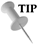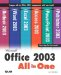# Inserting a Range Name into a Formula or Function

### Inserting a Range Name into a Formula or Function

As previously discussed in this lesson, range names make it easy for you to specify a range of cells in a formula or function. To insert a range name into a formula or function, follow these steps:

1. Click in the cell where you want to place the formula or function.

2. Type the formula or function (begin the formula or function with the equal sign).

3. When you are ready to insert the range name into the formula or function, select the Insert menu, point at Name , and then select Paste . The Paste Name dialog box appears (see Figure 11.4).

##### Figure 11.4. Use the Paste Name dialog box to insert a range name into a formula or function.4. Select the range name you want to place in the formula or function, and then click OK .

5. Finish typing the formula or function (including the appropriate operators).

6. Press Enter to place the formula or function into the cell and return the calculated value.Type the Name of a Range When you are creating a formula or function using a range name and you remember what that range name is, you can type it into the formula or function. You don't have to use the Paste Name dialog box.Microsoft Office 2003 All-in-One
ISBN: B005HKSHB2
EAN: N/A
Year: 2002
Pages: 660
Authors: Joe Habraken

Similar book on Amazon

flylib.com © 2008-2017.
If you may any questions please contact us: flylib@qtcs.net